# Random Math Stuff

## Prime Numbers

3.22.2022

List of prime numbers:

Prime Number Checker:

## "Prinary" Numbers

3.22.2022

Each natural number can be written in binary, as unique sums of powers of 2 (14 = 1110). This translates to 14 = (0×20) + (1×21) + (1×22) + (1×23), or just 21 + 22 + 23.

Because each number is uniquely expressed in binary, the set of these powers of two, {1, 2, 3}, uniquely represents 14. If we correspond each power of 2 to a prime number, we can multiply them together to guarantee a unique number gets mapped to each number. We'll say 0 corresponds to the 1st prime number, 1 corresponds to the 2nd, 2 to the 3rd, and so on. So {1, 2, 3} for the number 14 corresponds to (2nd prime)×(3rd prime)×(4th prime), which is 3×5×7 which equals 105. It's important to note that "105" is the natural number "105", not the string "1", "0", "5".

If we use this rule for every number, we can generate a list of the so-called "prinary" numbers (prime binary) for each number.

n written in prinary (starting with n = 0): (OEIS A019565)

Type number to convert to prinary:
Interesting Properties of Prinary Numbers:
• Prinary numbers are unique. That is, each prinary number maps to one and only one natural number (e.g., 38 = 195 in prinary, so no other number will be 195 in prinary).

## Higher Dimensional Triangular Numbers

3.22.2022

Triangular numbers are numbers that can be formed into triangles. The n-th triangluar number is the sum of the first n natural numbers. This means:

1st Triangular Number = 1
2nd Triangular Number = 1 + 2 = 3
3rd Triangular Number = 1 + 2 + 3 = 6
4th Triangular Number = 1 + 2 + 3 + 4 = 10,
and so on.

This can be generalized into higher dimensions, such as the tetrahedral numbers, which are the 3D equivalent of the triangular numbers. Similar to the triangular numbers, the n-th tetrahedral number is the sum of the first n TRIANGULAR numbers, instead of the first n natural numbers.

This applies to any dimension--the 4D triangular numbers are found by adding the first n 3D triangular numbers, the 65D triangular numbers are found by adding the first n 64D triangular numbers, and so on. This applies backwards as well, the 1D triangular numbers are just the natural numbers 1, 2, 3, etc., and the 0D triangular numbers are just all 1, 1, 1, ...

Slide to change the dimension:

2-dimensional triangular numbers:

## Uniform Modular Covers

3.23.2022

A natural number s is said to be a "uniform modular cover" (UMC) of n if s is coprime with n and less than n. Being a uniform modular cover means that you can continually add s to 0 (mod n) and eventually hit every number within the ring of integers modulo n. A visualization of the number 3 being a uniform modular cover of 10 is shown below: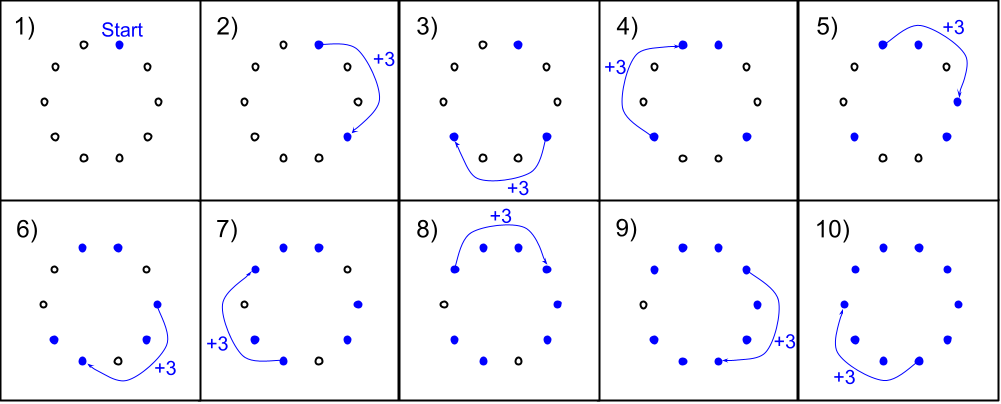There are 10 positions within mod 10 (0, 1, 2, 3, 4, 5, 6, 7, 8, 9), and starting at any position and continually adding 3 will eventually reach every position, without repeating. This works because 3 is coprime with 10; for example, 4 would not be a UMC of 10, because they share the factor 2. Here is the path of positions that would be reached by continually adding 4 within the modulo group for 10 (meaning we "wrap around" after reaching 10)

Start: 0
+4 = 4
+4 = 8
+4 = 2
+4 = 6
+4 = 0

And now we ended back where we started at position 0, so the entire path will repeat forever, but we haven't touched every number. This means 4 is NOT a UMC of 10. The uniform modular covers of 10 are {1, 3, 7, 9}. All of these will reach all 10 position of the integers modulo 10 if continually added from a starting position; all other numbers will not. This is because 1, 3, 7, and 9 are the only numbers less than 10 that are coprime with 10.

The quantity of UMC's that each number has ends up being Euler's totient function (count of numbers less than or equal to n that are coprime with n).

Number of uniform modular covers of n (also Euler's totient function): (OEIS A000010)

Interesting Properties of Uniform Modular Covers:
• Every number except 0 and 1 has an even number of UMCs
• Every prime number p has exactly p - 1 UMCs, because every number less than a prime number is coprime with it

## Perfect Modular Stars

3.23.2022

The idea of a number being a "perfect modular star" is directly related to the idea of uniform modular covers from the previous section. A number n is a perfect modular star if and only if floor((n - 1) / 2) is a uniform modular cover of n. This can be interpreted as: if the closest whole number to half of n (excluding exactly half of n) is coprime with n (rounded down).

For example: 10 is a perfect modular star if 4 is coprime with 10 (4 is the closest number to 5 that is not 5, rounded down). For an odd number example, 19 is a perfect modular star if 9 is coprime with 19 (9 is the closest number to 9.5, which is half of 19, rounded down). 10 is NOT a perfect modular star because 4 is not coprime with 10, and 19 IS a perfect modular star because 9 is coprime with 19.

The connection to the idea of "stars" can be seen with a star diagram: a path (like the ones taken in the "Uniform Modular Covers" section) is taken around a circle by taking uniform steps, and with lines tracing out the connected positions. The following star diagram shows the 2-path mod 10 (starting at position 0 and adding 2, mod 10):This demonstrates how 2 is NOT a uniform modular cover of 10, since not every point is touched before it repeats. To contrast a perfect modular star with a non-perfect modular star, two paths are shown: The first is the 3-path mod 10 (adding 3 to a ring of 10), and the second is the 7-path mod 16 (adding 7 to a ring of 16).Both of these are uniform modular covers, but only 16 is a perfect modular star because it's step size of 7 is closest to being directly opposite each point. In other words, adding 7 to the mod 16 circle is very close to directly jumping across, whereas adding 3 to the mod 10 circle is more like walking around the edge. The arms are pointier, and the center shape where the lines intersect is smaller. Also, the simplest star shape that everyone is familiar with, the 5-pointed star, is an example of a perfect modular star.

List of numbers that are perfect modular stars: (OEIS A107750)

List of numbers that are NOT perfect modular stars: (OEIS A016825)
Interesting Properties of Perfect Modular Stars:
• Every prime number is a perfect modular star
• Every number of the form 2p + 2 is a perfect modular star (with p as a prime number, excluding p = 2). For example, 16 can be written as 2(7) + 2, and is therefore a perfect modular star.
• Every number that is 2 times an odd number will NOT be a perfect modular star--and every other number will be (excluding 2×1, as 2 is considered a perfect modular star).
• Because every NON-perfect modular star can be written as 2 times an odd number, and odd numbers can be written as 2n + 1, every non-perfect modular star can be written as 2(2n + 1), or 4n + 2. This makes it clear that every non-perfect modular star will be congruent to 2 (mod 4). Therefore, a number is a perfect modular star if, and only if, it is NOT congruent to 2 (mod 4).

## Riffle Periods

3.25.2022

A mathematically perfect riffle of a deck of cards is defined as alternating cards from the top half of the pile and the bottom half of the pile, to create a new shuffled deck. A visualization is shown below.After a certain number of riffles, the deck will return to its original order. In the above example, this will happen after 4 riffle shuffles. This means the so-called "riffle period" for 6 is 4.

A standard deck of 52 cards turns out to have a riffle period of merely 8. This means that if you take a deck of cards and perfectly riffle it 8 times, it will end up in the exact same original order. Interestingly, if you increase the size up to 53 cards, it takes a full 52 riffles to have it return to its original order.

Riffle period of a deck of size n: (OEIS A024222)

Type number to calculate the riffle period of:
Riffle Period:
8

One interesting property of riffle periods is that every odd number and the number after it always have the same riffle period (e.g., 43 and 44 both have a riffle period of 14). Because of this, a more interesting sequence might be one where only every other number is considered (removing the unnecessary duplicates).

Riffle period of a deck of size 2n+1: (OEIS A002326)

Related Concept: "Unwieldy Deck Numbers"

Unwieldy Deck Numbers are numbers such that their riffle period is one less than themselves. For example, 53 is an Unwieldy Deck Number because it has a riffle period of 52. I call these numbers "Unwieldy Deck Numbers" because as a deck, it is awkward and complicated to manipulate it to its original state with riffles.

Unwieldy Deck Numbers (each number n has a riffle period of n - 1): (seems to be OEIS A050229)

Related Concept: "Highly Rifflic Numbers"

Another interesting property of the riffle period sequence is that it is not monotone increasing, and jumps around constantly. “Highly rifflic numbers” are then of interest, defined as a number with a higher riffle period than any number lower than it. For example, 25 is a highly rifflic number because it has a riffle period of 20, and no number below 25 has a riffle period higher than 20.

Highly Rifflic Numbers (each number n has a higher riffle period than any number less than n): (OEIS A139099)

It is important to note that the numbers in this sequence are the numbers whose riffle periods are record values, not the riffle periods themselves. So the presence of 121 in the sequence is because the riffle period of 121 is 110, and 110 is greater than the riffle period of any number smaller than 121. The “complement” of this sequence would be the sequence of the actual riffle periods of each highly rifflic number, which is as follows:

Riffle Periods of Highly Rifflic Numbers:

Another interesting fact about highly rifflic numbers is that a highly rifflic number n has a riffle period of 2n-2. I can't prove this, but it holds for the first 2,000 riffle periods. The following image shows a plot with the y-axis showing the riffle period of the x-axis. The blue line is the line y = 2x - 2, and all highly rifflic numbers I have looked at have lied on this line.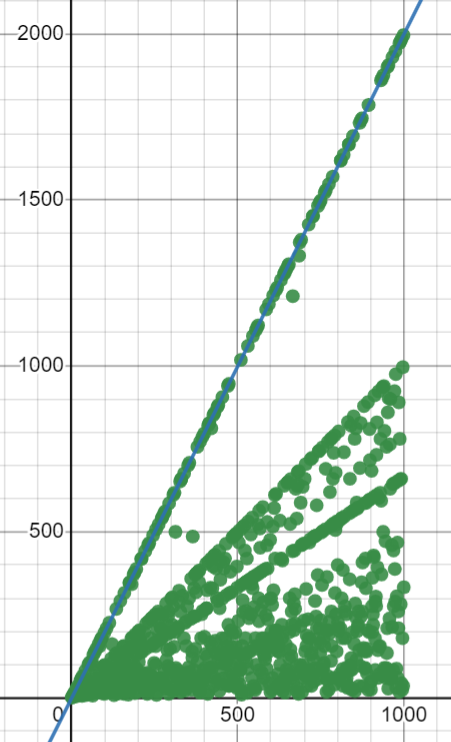Related Concept: Difference of HRN and UDN

Another interesting fact is that every unwieldy deck number is a highly rifflic number, so looking at the difference of the two sets could prove interesting (Highly Rifflic minus Unwieldy). I don't have a short name for it, but "Highly Rifflic but not Unwieldy Numbers" are number `n` that have a higher riffle period than any number below them, but their riffle period is not `n - 1`. Very interestingly, there are only 5 of these numbers I have found, and they are all squares...

Highly Rifflic but not Unwieldy Numbers: (Difference of HRN and UDN)

## The Natural Continued Fraction

2.16.2023

The Natural Continued Fraction is defined by this infinite continued fraction: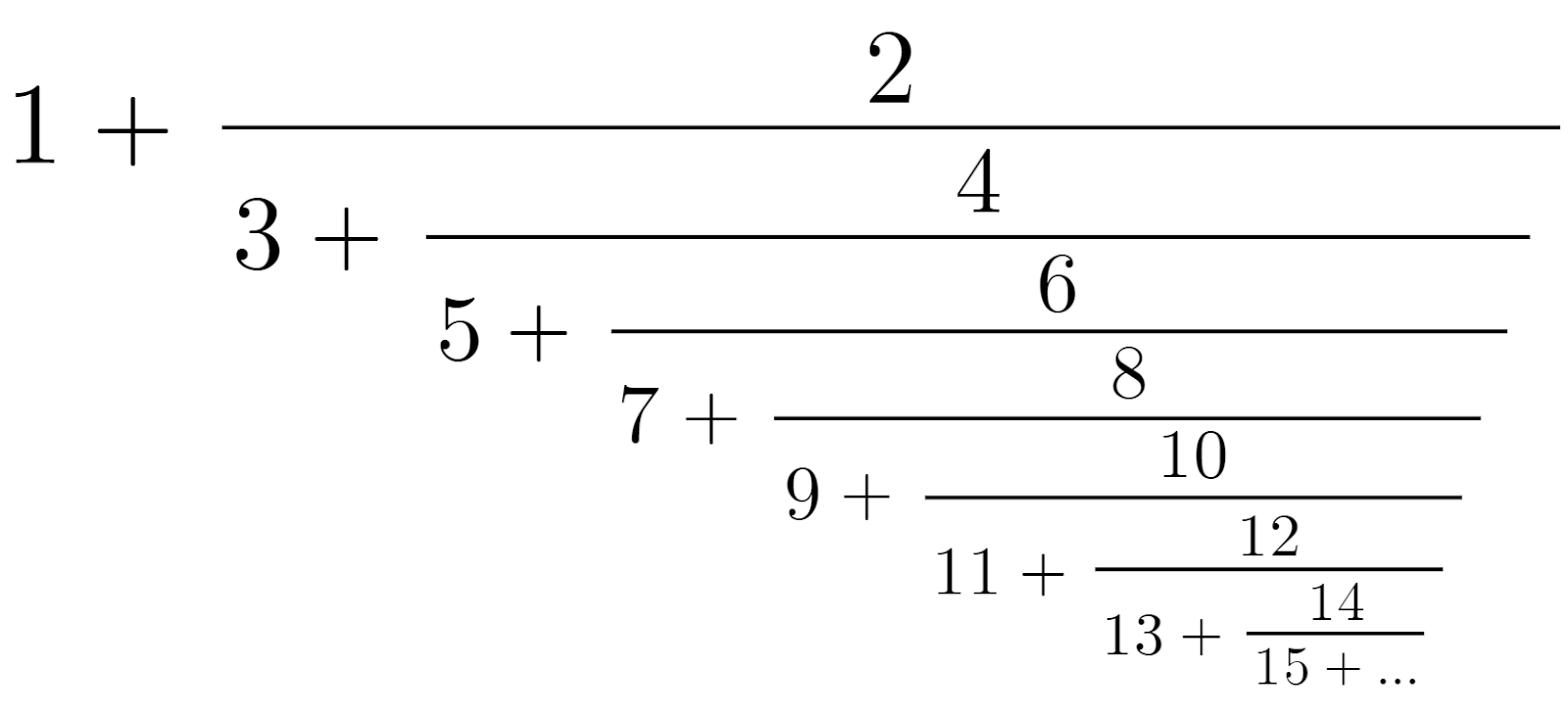The name comes from the fact that the coefficients of this fraction are the natural numbers (1, 2, 3, ...). The reason this is interesting is that most continued fractions that pop up in math have seemingly random coefficients, such as the fraction for pi, whereas the Natural Continued Fraction has coefficients that are simply the natural numbers.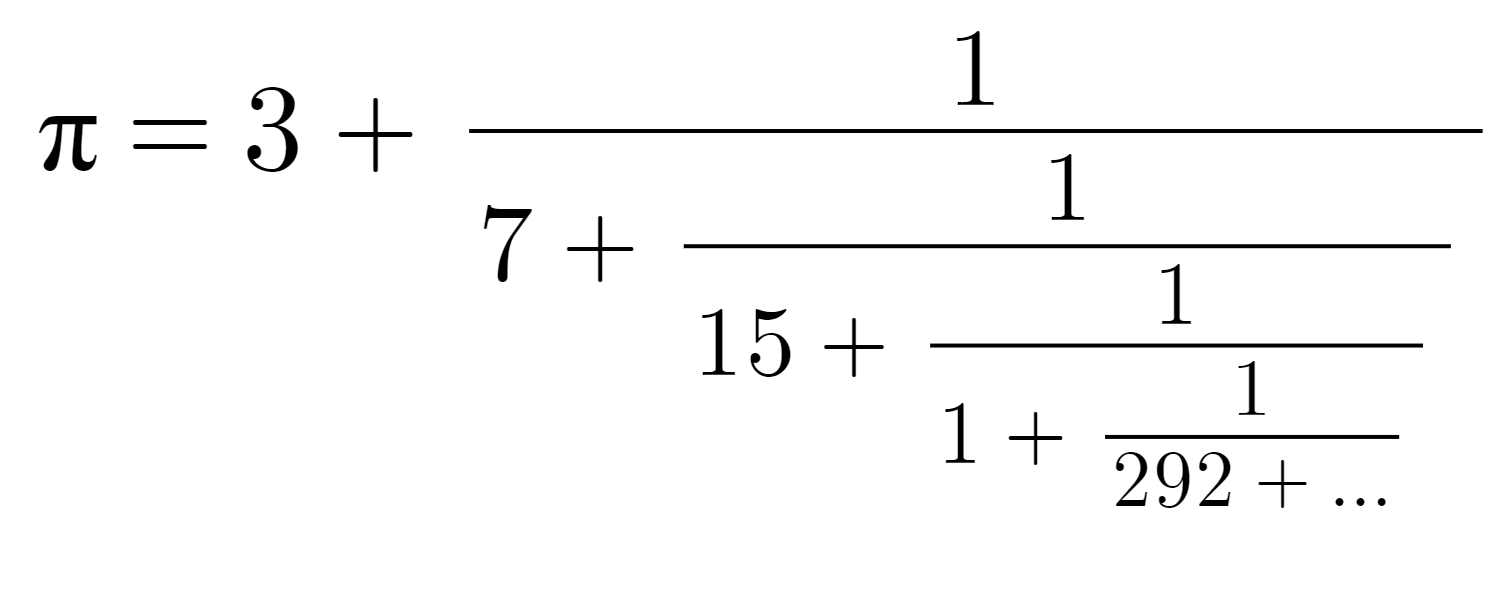As more and more terms are appended, the decimal expansion of the Natural Continued Fraction approaches this value: (Sequence A113011 in the OEIS)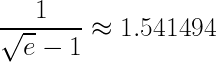We can still find a different sequence within this number, however, by taking the convergents of the series. We obtain each convergent by cutting off the fraction at the next number, so we obtain the following sequence of sums: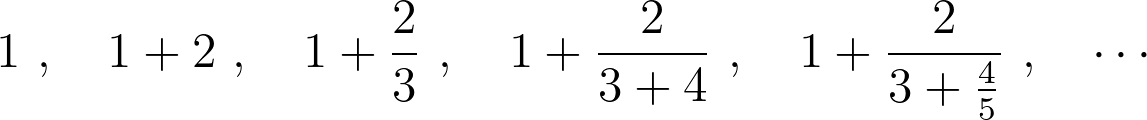If we simplify the fractions at every step, we obtain a series of fractions that begins with 1, 3, 5/3, 9/7, etc.

The n-th convergent of the Natural Continued Fraction:
TODO: Write function to calculate this